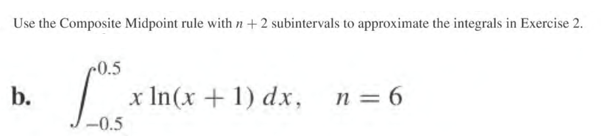# Question Solved1 AnswerUse the Composite Midpoint rule with n +2 subintervals to approximate the integrals in Exercise 2. 0.5 b. x In(x + 1) dx, n = 6 -0.5Transcribed Image Text: Use the Composite Midpoint rule with n +2 subintervals to approximate the integrals in Exercise 2. 0.5 b. x In(x + 1) dx, n = 6 -0.5
More
Transcribed Image Text: Use the Composite Midpoint rule with n +2 subintervals to approximate the integrals in Exercise 2. 0.5 b. x In(x + 1) dx, n = 6 -0.5